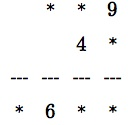(1) Some 4 digit numbers can be written as the product of a 3 digit number and a 2 digit number using the digits 1 to 9 each once and only once.

Can you use the digits 1 to 9 once, but only once, to replace the stars and complete the multiplication  * *9  x  4*  = * 6 * * ?

(Hint: what is the largest 3 digit number you could use?)

(2) The next funny factorisation is 186 x ** = **** and again you must replace the * symbols so that the digits 1 to 9 each appear once and only once.

(3) The number 4396 can be written as a product in this way. Can you find the factors? (Hint: first find the prime factors of 4396.)

(4) Maths is full of surprises! The number 5796 can be written as a product like this in two DIFFERENT ways, and so can the number 5346. Can you find these four funny factorisations?

There are only seven possible funny factorisations? Can you find them all?

See the Notes for Teachers – Funny Factorisation and more about the problem on the  NRICH website.

Tagged with:

### 5 Responses to Funny Factorisation

1.Nelly Malamlela says:

I have no idea can you help me?

2.Buyi Taho says:

1)I used trial and error to get this 139×41=56992
2) 186 x21=3906
Im not sure where is the restriction of not repeting a number, is it in the answer or while multiplying?

3.toni says:

Buyi, you must read the question carefully. If you replace the * symbols with digits in

* * 9 x 4 * = * 6 * *

you have 9 digits and the digits 1 to 9 must each appear once and only once.

There is no point in putting answers in these comment boxes. You can click on the ‘Notes for Teachers’ link and see the answers there. These comment boxes are for discussing how you use the problems in your lessons.

4.Pele Pele says:

Presented the problem to my grade 10 when introducing the term factorization,they got difficulties on getting the problem but do understant the concept and processes around it.Multiplication skills are needed for factorization

5.Ntholeng Lekhetha says:

I took out 149 and 169 out as 4 and 6 already there. I started multiplying 41, 42, 43, 45, 48 by 129, 139, 159
.159 x 48 =7632
I got it before they multiply by 179 and 189.